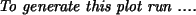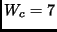Next: 5.1.2 Integration using hackcode1 Up: 5.1 N-body experiments Previous: 5.1 N-body experiments   Contents   Index

## 5.1.1 Setting it up

In Chapterwe already used mkplummer to create a Plummer model; here we shall use the program mkommod ("MaKe an Osipkov-Merritt MODel") to make two random N-body realizations of a King model with dimensionless central potentialand 100 particles each. The small number of particles is solely for the purpose of getting results within reasonable time. Adjust it to whatever you can afford on your CPU and test your patience (see Appendixbenchmarks).

    1% mkommod in=$NEMODAT/k7isot.dat out=tmp1 nbody=100 seed=280158  These models are produced in so-called RMS-units in which the gravitational constant G=1, the total mass M=1, and binding energy E=-1/2. In case you would like virial units (E=-1/4)5.2the models have to be rescaled using snapscale:  2% snapscale in=tmp1 out=tmp1s rscale=2 "vscale=1/sqrt(2.0)"  In the case that your user interface was not compiled with the NEMOINP5.3directive, the vscale expression has to be calculated by you, i.e. vscale=0.707107. Also note the use of the quotes in the expression, to prevent the shell to give special meaning to the parenthesis, which are shell meta characters. The second galaxy is made in a similar way, with a different seed of course:  3% mkommod in=$NEMODAT/k7isot.dat out=tmp2 nbody=100 seed=130159


This second galaxy needs to be rescaled too, if you want virial units:

    4% snapscale in=tmp2 out=tmp2s rscale=2 "vscale=1/sqrt(2.0)"


We then set up the collision by stacking the two snapshots, albeit with a relative displacement. The program snapstack was exactly written for this purpose:

    5% snapstack in1=tmp1s in2=tmp2s out=i001.dat \
deltar=4,0,0 deltav=-1,0,0


The galaxies are initially separated by 4 unit length and approaching each other with a velocity consistent with infall from infinity (parabolic encounter). The particles assembled in the data file i001.dat are now ready to be integrated.

To look at the initials conditions we could use:

    6% snapplot i001.dat xrange=-5:5 yrange=-5:5

which is displayed in Figure.Next: 5.1.2 Integration using hackcode1 Up: 5.1 N-body experiments Previous: 5.1 N-body experiments   Contents   Index
(c) Peter Teuben